# Basic Commands, Variables, and Data Types

There are many commands in DarkBASIC; some are very easy to understand, but many are more complicated. To understand those commands, you will need to first cover some fundamental groundwork. Programming is more than just knowing all the commands. You need to understand how to use variables and learn the basic data types. When you have mastered the information in this chapter, you will be on the right track for writing full-blown programs in DarkBASIC.

This chapter explains everything you need to know about variables—what they are, how they are defined, and how they are used. Data types are next. There are three data types to cover—integer, decimal, and string. After I lay the groundwork, I will cover a few basic commands. At the end of this chapter, you will be on your way to writing entire programs, and I will show you several examples along the way.

## Understanding Variables

I briefly mentioned variables in the last chapter (enough to tantalize you?), but this chapter is dedicated to the subject. One might go so far as to claim that variables are the foundation of computer programs. They provide a means to store data in a program. A variable is stored in memory and accessed through its name, like this:

```HugeNumber = 3828549
ShoeSize = 12
Person\$ = "Carrie-Anne Moss"
```

As you can see, variables can store numbers or words (also called strings).

### What Is a Variable?

In the old days when programmers used machine and assembly language, they would create variables by simply grabbing a chunk of memory and then storing a number in the newly acquired spot. This was called memory allocation, which is a valid term in software today. Reserving a space in the computer's memory was a manual process that required the programmer to keep track of exactly where that space was located by creating a pointer—from which variables were derived.

Assembly language is a very low-level programming language that uses mnemonic (symbols that are easy to remember) instructions to represent the machine language instruction set of the computer itself—actually, the microprocessor in the physical sense. These mnemonic instructions allow the programmer to perform mathematical, logical, memory, and input/output instructions using English words rather than pure binary, so Add might be used rather than 00101010. As you can imagine, it is very difficult to write programs at this level even with the mnemonics, so assembly is normally only used to write timecritical high-performance code, such as device drivers, graphics algorithms, and other system programs.

To make the task easier, programmers developed assembly language, made up of words and symbols that are very closely related to machine instructions but are much easier to write. Assembly permitted the use of mnemonic words to replace the specific addresses in the memory where the information was stored. Rather than keep track of a pointer's address, which in turn pointed to another address in the memory where actual data was located, a mnemonic was used as the pointer. Mnemonics are easier to remember than physical addresses in memory, so this made writing programs much easier. Here is an example:

```MOV AX, 03h
INT 33h
```

Those two commands together tell the computer to get the mouse position. As you might imagine, assembly is difficult to master, and beginners always have an interrupt reference book handy. The first line moves the number 3 into the AX register. Registers are sort of like the piston chambers of a processor. Put something in the chamber and fire off an interrupt, and something will happen (only in this case, it is a number instead of a spurt of gasoline and air).

The second line is an interrupt call—the spark plug of assembly language. There are many interrupts in assembly that do all kinds of weird things, such as polling the mouse. Another popular old MS-DOS interrupt is INT 21h, which was very common in the old days when games were developed for MS-DOS (before Windows or DirectX came along).

The AX in the first line is the assembly equivalent of a variable, although that is a bit of a stretch because AX is actually a physical part of the processor. If you were to take a processor and look at it through a microscope, you would theoretically be able to locate that AX register amidst a tight cluster of registers in the core of the chip.

Over time, assembly language and all the difficult-to-remember mnemonic words such as MOV and INT were replaced by more advanced languages that were closer to human language. DarkBASIC is what you might call an ultra high-level language because it has so many built-in features. In contrast, assembly language is extremely low-level because it is closer to machine language in form. Fortunately for us, DarkBASIC keeps track of all the variables in a program, including the type of data stored in variables.

### Variable Notation

So how do you define a variable? You simply give it a name. There are rules for defining a variable name. You cannot start a variable with a number or punctuation mark, and you cannot have a space in the name. Other than that, the sky is the limit. For example, if you want to store the number of shots fired from a gun, you would define the variable like this:

```ShotsFired = 5
```

You might also want to store how many shots are in the gun. To do so, just define another variable the keeps track of that value.

```ShotsInGun = 6
```

Performing Basic Math Operations with Variables

You can also perform math functions on variables, including the four basic mathematical functions—addition, subtraction, multiplication, and division. Addition problems use the plus sign (+), subtraction problems use the minus sign (−), multiplication problems use the asterisk (*), and division problems use the forward slash (/). Here is a short sample program called MathExample1, which demonstrates how to use the basic math operators. You can open this program from the CD-ROM or type it into DarkBASIC yourself. Figure 3.1 shows the output of MathExample1.Figure 3.1: The MathExample1 program demonstrates basic math operations.

```REMSTART
---------------------------------
Beginner's Guide To DarkBASIC Game Programming
Copyright (C)2002 Jonathan S. Harbour and Joshua R. Smith
Chapter 3 - MathExample1 program
---------------------------------
REMEND

REM the variable "Answer" will be equal to 4

REM the variable "Answer" will be equal to 3

REM the variable "Answer" will be equal to 4

REM the variable "Answer" will be equal to

REM wait for a key press
WAIT KEY
END
```

Using More Than One Variable in a Formula

Now, here's a trick: Instead of using plain numbers (which are called literals in computer-speak) in a variable, you can use other variables. For example, to calculate the speed at which an object is traveling (such as your car), you need a variable for distance and one for time. The speed is just distance divided by time. The following program, called MathExample2, shows how to solve this simple problem with DarkBASIC. Figure 3.2 shows the output of MathExample2.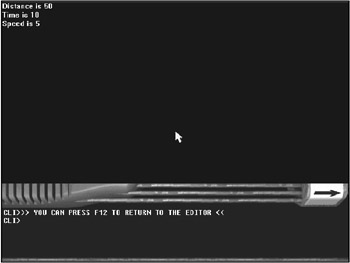Figure 3.2: The MathExample2 program demonstrates how to use variables in a calculation.

```REMSTART
---------------------------------
Beginner;s Guide To DarkBASIC Game Programming
Copyright (C)2002 Jonathan S. Harbour and Joshua R. Smith
Chapter 3 - MathExample2 program
---------------------------------
REMEND

REM create some variables
Distance = 50
Time = 10
Speed = Distance / Time
PRINT "Distance is "; Distance
PRINT "Time is "; Time
PRINT "Speed is "; Speed

WAIT KEY
END
```

Order of Operations

In DarkBASIC, mathematical operations have an order to them. Division and multiplication are performed first, followed by addition and subtraction. You can bypass that order if necessary by enclosing your problem in parentheses. Parentheses tell DarkBASIC to evaluate a certain part of the problem first. For example, in the equation 2 * 3 + 5, the answer is determined by first multiplying 2 by 3 and then adding 5, which equals 11. Imagine if you were to first add 5 to 3 and then multiply by 2. The answer would be 16, which is incorrect. By using parentheses, though, you can force DarkBASIC to put priority on part of the calculation. 2 * (3 + 5) = 16. The following program, called MathExample3, demonstrates the use of parentheses. Figure 3.3 shows the output of MathExample3.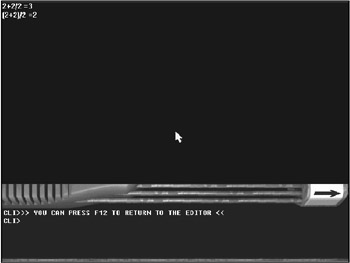Figure 3.3: The MathExample3 program demonstrates how to use parentheses to affect the order of operations in a math formula.

```REMSTART
---------------------------------
Beginner's Guide To DarkBASIC Game Programming
Copyright (C)2002 Jonathan S. Harbour and Joshua R. Smith
Chapter 3 - MathExample3 Program
---------------------------------
REMEND

REM this will evaluate to 3
Answer = 2 + 2 / 2
PRINT "2 + 2 / 2 ="; Answer

REM this will evaluate to 2
Answer = (2 + 2) / 2
PRINT "(2 + 2) / 2 ="; Answer

WAIT KEY
END
```

This is known as order of operations. The only way to bypass this order is by using parentheses. Let me throw a few more problems at you; see if you can figure them out. Use Table 3.1 if you need a guide to the order of operations.

1. 1. 4 + 3 / 3
2. (4 + 4) / 2
3. 4 + 4 * 2
4. 4 * (4 + 4)

The answers to these problems are

1. 5
2. 4
3. 12
4. 32
Table 3.1: Order of Operations

Operator

Precedence

Description

( )

First

Parentheses to set precedence

*, /

Second

Multiplication and division

+, -

Third

It's pretty complicated to keep the order of things in mind, especially when you are trying to write a program to solve a math problem for an algebra or calculus class! Table 3.1 lists the basic mathematical operations and the order in which they are evaluated.

### Variable Scope

Sometimes when you examine a problem, you need to determine its scope … that is, you need to determine how far the problem extends. Variables are similar in that they have a scope. There are two different types of scopes that variables obey—global and local.

Global Variables

Global variables are accessible anywhere in the program. It is like having a Palm Pilot in your pocket on the bus. Although you don't have access to your home computer, you still have access to all your information via the Palm Pilot. Information in a global variable is accessible anywhere in the program. Global variables are declared at the top of your source code with the DIM command.

```DIM GlobalVariable(1)
```

Local Variables

Local variables are a different story. They are only visible (or available) in the current subroutine in which they reside. (I will describe subroutines in more detail in Chapter 6, "Making Programs Think: Branching Statements and Subroutines.") Figure 3.4 illustrates the difference between local and global variables. In this figure, the main program has global variables, while a subroutine has local variables (in other words, variables that have scope only within the subroutine). The subroutine can use the global variables, but the main program cannot use the variables inside the subroutine.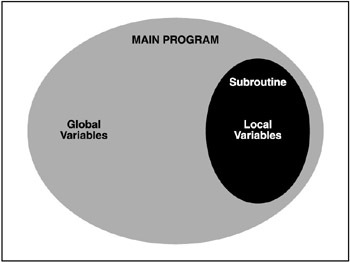Figure 3.4: The difference between local and global variables

Your home computer is a good analogy to explain global and local variable scope. That's right; just think of your computer in light of this discussion. You have all kinds of information on it, such as your e-mail, photos, school or work assignments, and letters to friends. You can only access the files on your computer when you are at home and the computer is turned on. Or, suppose you are sharing files on your computer over the Internet, using something like an FTP (File Transfer Protocol ) server or Web server. The FTP or Web files might be open to the public, but all of the other files on your computer that are not shared will not be visible. That relationship is similar to the local/global scope relationship.

### Creating Global Variables Using the DIM Command

A variable generally stores one value at a time. Sometimes one value is just not enough. For example, you might want to keep track of ten different answers instead of one. You can extend the scope of a variable to encapsulate ten values by using the DIM command. DIM tells DarkBASIC to create an array.

An array is an area of memory reserved for a sequential list of values of the same data type (such as integers or strings).

You can then use the multiple values assigned to your variable. You can reference each value by either a number or another variable. Here's another sample program, called ArrayExample, which shows you how to use two arrays in a program (see Figure 3.5). You will find this program on the CD in the SourcesChapter03ArrayExample folder.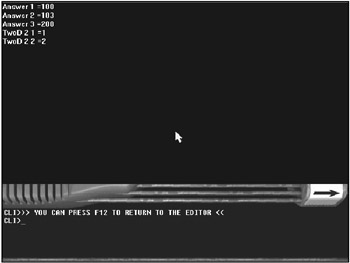Figure 3.5: The ArrayExample program demonstrates how to use arrays.

```REMSTART
---------------------------------
Beginner's Guide To DarkBASIC Game Programming
Copyright (C)2002 Jonathan S. Harbour and Joshua R. Smith
Chapter 3 - ArrayExample Program
---------------------------------
REMEND

NextOne = 3

DIM TwoD(2,2)
TwoD(2,1) = 1
TwoD(2,2) = 2
PRINT "TwoD 2 1 ="; TwoD(2,1)
PRINT "TwoD 2 2 ="; TwoD(2,2)

WAIT KEY
END
```

Arrays can have more than one dimension. Kind of like the Twilight Zone: "You've entered a new dimension of sight and sound… ." The best way to visualize an array is to imagine a row of squares. While the row is an entity itself, you can identify each square in the row individually as well. To get to a particular square in a row, you reference that square by its position. Arrays in DarkBASIC can be stored five dimensions deep, which is far more than you will ever need. (If you ever do need more than five dimensions, you might need to redesign the logic for your program.) Figure 3.6 illustrates a two-dimensional array with ten columns and seven rows of boxes (which results in 70 elements in this array).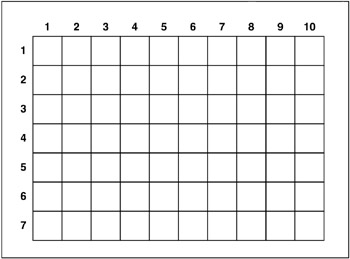Figure 3.6: A two-dimensional array can be illustrated with columns and rows of boxes, like the cells in a spread-sheet program.

## Understanding Data Types

Up to this point, I have been talking about variables and arrays, and how you can store values in them. I've shown you some illustrations to help you to understand the concepts. Now I would like to get into more detail by explaining the different types of information that you can store in a variable. In computer-speak, this is called the data type. I will explain each data type shortly; in the meantime, Table 3.2 introduces the data types and the notations required to create them.

Table 3.2: Variable Types

Data Type

Notation

Example

Integer

None

NumberOfCars

Real

#

String

\$

Name\$

### What Is a Data Type

There are three types of data that make up the data types in DarkBASIC. While other programming languages (such as Visual Basic, C++, or Delphi) have many different data types, DarkBASIC keeps the list down to three items. As I have explained, DarkBASIC was designed specifically for writing games and graphics demos, and such programs don't often need the plethora of advanced data types available in other languages that were designed to solve programming problems (not specifically games, which is where DarkBASIC shines). There are some differences in this regard between DarkBASIC and DarkBASIC Professional, in that the latter supports many more data types than the former. I will reserve the discussion of the more advanced data types in DarkBASIC Pro for a later chapter. For now, an introduction to the basics is essential.

### Basic Data Types

There are three basic data types—integer, decimal, and string. Each type is used in a special way to let DarkBASIC know what type of data a certain variable needs to store. For example, decimal variables (which keep track of floating-point or real numbers) must be referenced specifically with the pound sign (#) following the variable name. For example, Num# = 0.5 declares a variable called Num, gives it a decimal data type, and then sets the value to 0.5. In a sense, you might think of a variable's data type as a type of storage bin, such as the bins in the fruit and vegetable aisle at your local grocery store. There are separate bins for carrots, lettuce, potatoes, onions, peaches, apples, bananas, and so on. You would not want the fruits and vegetables to be piled together into a single large bin because that would make it difficult to sort it out and find what you want. The same theory applies to the way DarkBASIC keeps track of variables by identifying the type of value stored in each data type.

Integers

An integer can hold whole numbers. That is to say, an integer is a number that is not a fraction. 5.5 is not an integer because it has a fraction part, but 5 is. The following variables are integers.

```A = 1
clicks = 5
counter = 0
scoredifference = -5
```

Integers can be positive or negative. To make a variable negative, just add a minus (−) sign in front of the value. Following is a sample program called IntegerExample, which demonstrates how to use integers (see Figure 3.7).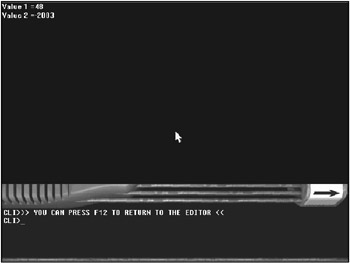Figure 3.7: The IntegerExample program demonstrates how to use integers.

```REMSTART
----------------------------------------
Beginner's Guide To DarkBASIC Game Programming
Copyright (C)2002 Jonathan S. Harbour and Joshua R. Smith
Chapter 3 - IntegerExample Program
----------------------------------------
REMEND

REM example of integers
Value1 = 48
Value2 = -2003
PRINT "Value 1 ="; Value1
PRINT "Value 2 ="; Value2
WAIT KEY
```

Decimals

A decimal can store a fraction; it is also called a real number. To define a variable as a decimal, add a # sign after the variable name. DarkBASIC will then know to use that variable as a decimal and as an integer. The following variables are real numbers.

```PI# = 3.14159
xpos# = 5.332
ypos# = -1.334
zpos# = 2.234
```

Real numbers can be positive or negative; just like with integers, you simply add a minus (−) sign in front of the value to make it negative. The DecimalExample program, shown in Figure 3.8, demonstrates how to use floating-point variables in DarkBASIC. You will find this program on the CD in the SourcesChapter03DecimalExample folder.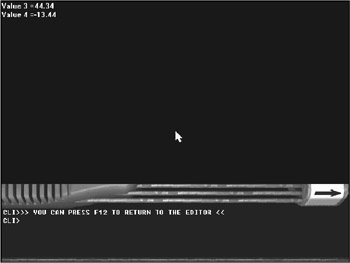Figure 3.8: The DecimalExample program demonstrates how to use decimals.

```REMSTART
---------------------------------
Beginner's Guide To DarkBASIC Game Programming
Copyright (C)2002 Jonathan S. Harbour and Joshua R. Smith
Chapter 3 - DecimalExample Program
----------------------------------
REMEND

REM example of integers
Value3# = 44.34
Value4# = -13.44
PRINT "Value 3 =";
PRINT Value3#
PRINT "Value 4 =";
PRINT Value4#
WAIT KEY
```

Character Strings

A string is literally a string of characters that is not interpreted as a number. This means you can store any characters you wish in a string—including numbers, letters, symbols, and punctuation. Any numbers stored as part of a string are treated just like letters and punctuation marks and are just symbols as far as DarkBASIC is concerned. To define a variable as a string, add a \$ sign after the variable name. All string values must be enclosed in quotes (""), which tell DarkBASIC where your string starts and ends. The following variables are strings.

```MyName\$ = "Dirk Gentry"
FirstFiveLetters\$ = "ABCDE"
LastFiveLetters\$ = "VWXYZ"
```

A string can also be blank, or what's called the empty string. To create an empty or blank string, you initialize the string by setting it equal to "". (Note that there is nothing between the quotes.) Figure 3.9 shows the StringExample program, which demonstrates how to use strings in DarkBASIC.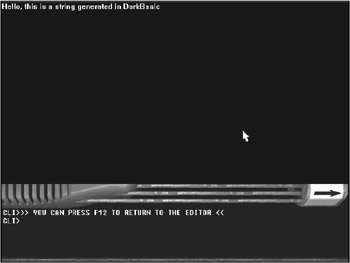Figure 3.9: The StringExample program demonstrates how to use strings.

```REMSTART
---------------------------------
Beginner's Guide To DarkBASIC Game Programming
Copyright (C)2002 Jonathan S. Harbour and Joshua R. Smith
Chapter 3 - StringExample Program
---------------------------------
REMEND

REM example of strings
String1\$ = "Hello, this is a string generated in DarkBASIC"
String2\$ = ""

PRINT String1\$
PRINT String2\$
WAIT KEY
```

### Converting Data Types

Sometimes you will need to convert a variable from one type to another. DarkBASIC provides the commands to do this. The STR\$ command will convert any variable type (usually integer or decimal) to a string. This is useful when you need to display a variable on the screen because there are some display commands that work only with strings.

```MyAge = 25
MyAgeString\$ = "This is my age "+str\$(MyAge)
```

The VAL command is the opposite of the STR\$ command; it converts a string into a number for use in a calculation or formula. There are many times when you will need to convert from one data type to another. The VAL command can be very handy because it ignores any spaces or tabs in a string when converting to a number (which is useful, for example, when you are reading values from a text file).

```MyAge\$ = "25"
MyAge = VAL(MyAge\$)
```

## Working with Basic Commands

Now is where the real fun begins. You are going to cover some basic DarkBASIC commands that will haunt you…er, be with you, for the rest of the book.

### The PRINT Command

PRINT is one of the most important commands in the DarkBASIC language. In Chapter 4, "Characters, Strings, and Text Output," you will learn some more variations of the PRINT command, but for now I want to give you the basics. The PRINT command takes a string as an argument. It looks like this:

```PRINT "text to display"
```

An argument is a variable or literal value that is passed to a subroutine or DarkBASIC command.

Notice that any text you want to output with the PRINT command is surrounded by quotes. That is so DarkBASIC knows what text you are asking it to print. If you want to add two different strings of text, you would do something like this:

```PRINT "Item1", "Item2"
```

Note that a comma separates each individual parameter. The comma actually adds a tab to the output. If you want to append one variable or text value to the end of the last one without the tab jump, you can use a semicolon (;) instead. Appending text is very useful in DarkBASIC when you want to display values on the screen, such as the high score in a game. The PrintExample program (shown in Figure 3.10) demonstrates how to use the PRINT command to display text followed by a variable.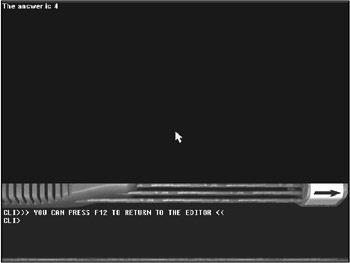Figure 3.10: The PrintExample program demonstrates how to use the PRINT command.

```REMSTART
---------------------------------
Beginner's Guide To DarkBASIC Game Programming
Copyright (C)2002 Jonathan S. Harbour and Joshua R. Smith
Chapter 3 - PrintExample Program
---------------------------------
REMEND

REM "The answer is 4" should be printed on the screen.
WAIT KEY
```
 Note I realize that some program listings are very short, but the sample programs in this chapter and the next few chapters are included on the CD-ROM for easy retrieval and execution. It's all part of the learning experience! Later chapters will assume that you are more familiar with the code, and I will not use code to explain every topic.

### The GET DATE\$ Command

Sometimes you will need the current date in a program. You might need this to store the last time a program was run or to print it on the screen. DarkBASIC has a GET DATE\$() command to retrieve the current computer date. Notice it has a \$ at the end, which means that GET DATE\$() returns a string. If you wanted to print the date, you would type the following code.

```PRINT "The date is ", GET DATE\$()
```

Did you notice that this command has a space between GET and DATE? DarkBASIC has some commands with spaces, which is somewhat difficult to comprehend at first, but once you have used them for a while, you will find these commands easy to remember.

The GET TIME\$ Command

Another useful command is GET TIME\$. You can use this command to keep track of how many hours you have been sitting at your computer programming in DarkBASIC. Notice that it's a string as well. All commands that give you information will return one of the three data types discussed earlier in this chapter.

```PRINT "The time is ", GET TIME\$()
```

### The EXECUTE FILE Command

The EXECUTE FILE command is slightly more complicated. It might not seem like much, but it is quite useful when you are writing a menu program. The EXECUTE FILE command runs any executable program (whether it is on a CD-ROM, the hard drive, or another accessible device or location) on your computer. I have written a program called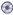sample.exe to use as an example.

The EXECUTE FILE command has three arguments. The first is the file name of a program to run (in this case,sample.exe). The ExecuteFileExample1 program (shown in Figure 3.11) demonstrates how to use the command.Figure 3.11: The ExecuteFileExample1 program demonstrates the EXECUTE FILE command.

This program, along with thesample.exe file, can be found on the CD-ROM in the SourcesDarkBASICCH03 folder. If you are using DarkBASIC Pro, the folder name is SourcesDBProCH03.

```REMSTART
---------------------------------
Beginner;s Guide To Game Programming With DarkBASIC
Copyright (C)2002 Jonathan S. Harbour and Joshua R. Smith
Chapter 3 - ExecuteFileExample1 Program
---------------------------------
REMEND

REM this will execute sample.exe
EXECUTE FILE "sample.exe", "", ""
```

The second argument that EXECUTE FILE accepts is a command-line parameter. This is the line that sends the arguments to the program you are trying to run. If you wanted to pass your name to thesample.exe program as a command-line parameter, you would add "your name" to the second parameter, as the ExecuteFileExample2 program demonstrates (see Figure 3.12).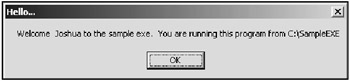Figure 3.12: The ExecuteFileExample2 program demonstrates how to pass a command-line parameter to a program that is being run.

You will find this program, along with thesample.exe file, on the CD in the SourcesChapter03ExecuteFileExample2 folder.

```REMSTART
---------------------------------
Beginner's Guide To Game Programming With DarkBASIC
Copyright (C)2002 Jonathan S. Harbour and Joshua R. Smith
Chapter 3 - ExecuteFileExample2 Program
---------------------------------
REMEND

REM this will execute sample.exe if it exists,
REM and add my name as an argument.
EXECUTE FILE "sample.exe", "Joshua", ""
```

The last argument used in EXECUTE FILE is the directory in which your program is located. This way DarkBASIC knows where to go to get the program for its data files. If nothing is specified here, the program will assume the current directory by default. If the program is not in the directory you specified, DarkBASIC will return an error. Figure 3.13 shows the output of the ExecuteFileExample3 program when the executable file can't be found.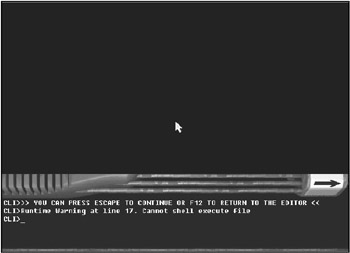Figure 3.13: The output of ExecuteFileExample3 shows that an error occurred.

```REMSTART
---------------------------------
Beginner's Guide To Game Programming With DarkBASIC
Copyright (C)2002 Jonathan S. Harbour and Joshua R. Smith
Chapter 3 - ExecuteFileExample3 Program
---------------------------------
REMEND

REM this will execute sample.exe if exists and
REM send the argument, Joshua and Force the
REM Working Directory to C:
EXECUTE FILE "sample.exe", "Joshua", "c:"
```

Notice how DarkBASIC generated an error. You can fix this if you just copysample.exe to C:. If you do, the result of the ExecuteFileExample3 program should look like Figure 3.14.Figure 3.14: The ExecuteFileExample3 program, successfully run

## Project

All right, now for a fun chapter project. When I was in school, I did some fun exercises in my typing class. I would draw pictures with the typewriter. I thought I would let you enjoy the same experience, only with DarkBASIC! Notice that I start the program with comments. If you would prefer to load the project instead of typing it all in, it is located on the CD in the folder called SourcesDarkBASICCH03Artist.

This program draws a picture of a smiley face, and it just happens to resemble a famous fellow involved in the DarkBASIC language (hint, hint!). It also contains variables to store your name and age so it can print them at the bottom of the picture. Once you have typed the program in, try modifying it to include your name and age. Creativity is key when writing games, even simple ones! Figure 3.15 shows the output of the Artist program.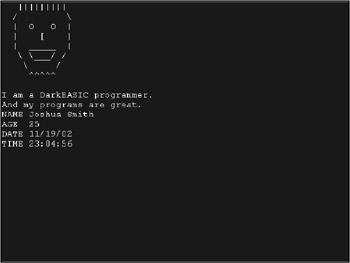Figure 3.15: This is what the Artist program looks like when it is run.

```---------------------------------
Beginner's Guide To DarkBASIC Game Programming
Copyright (C)2002 Jonathan S. Harbour and Joshua R. Smith
Chapter 3 - Artist Program
---------------------------------
REMEND

MyName\$ = "Joshua Smith"
MyAge = 25

SET TEXT FONT "Courier New"
SET TEXT SIZE 18

PRINT " ||||||||| "
PRINT " /  "
PRINT " | O O | "
PRINT " | [ | "
PRINT " | _____ | "
PRINT "  \___/ / "
PRINT "  / "
PRINT " ^^^^^ "
PRINT
PRINT "I am a DarkBASIC programmer."
PRINT "And my programs are great."
PRINT "NAME ", MyName\$
PRINT "AGE ", MyAge
PRINT "DATE ", GET DATE\$()
PRINT "TIME ", GET TIME\$()
WAIT KEY
END
```

## Summary

This chapter covered many of the fundamentals of programming. Data types, variables, variable scope (local and global), math formulas, and variable notation—these are all basic subjects, but they are the key elements that are important to master if you want to be a successful programmer. As you progress through the next few chapters, you will gain a broader understanding of the nuts and bolts of DarkBASIC programming that were introduced in this chapter.

## Quiz

The chapter quiz will help you retain the information that was covered in this chapter, and will give you an idea about how well you understand the subjects. Refer to Appendix A, "Answers to the Chapter Quizzes," to see how well you answered these questions.

 1 What is an argument in DarkBASIC? A fight you get in with the computer A virtual boxing match The information a command needs to be processed correctly The current time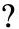2 What is an array in DarkBASIC? A science fiction concept An area of memory reserved for program data A PRINT statement None of the above3 What command defines a global variable? REMSTART PRINT DIM EXECUTE FILE4 Which is evaluated first in the order of operations? Things enclosed in parentheses Addition Multiplication Division5 What does the PRINT command do? Displays text on the screen Prints text to the printer Adds money to your virtual bank account Ends a program6 In EXECUTE FILE "jumpers.exe", "" is a valid command. True False, there is no EXECUTE FILE command False, there are not enough arguments False, there are too many arguments7 In DarkBASIC what does 2+2*2+6*(3+2) evaluate to? 17 46 36 148 Which is not a valid variable type? String Integer Real Caret9 Which command converts a string into an integer? STR\$() VAL() STRINGTOINTEGER() None of the above10 Which command prints the current date on the computer? PRINT GET TIME\$() PRINT GET DATE\$() PRINT WHATSTHEDATE() PRINT "Today"1 C 2 B 3 C 4 A 5 A 6 A 7 C 8 D 9 B 10 B

Part I - The Basics of Computer Programming

Part II - Game Fundamentals Graphics, Sound, Input Devices, and File Access

Part III - Advanced Topics 3D Graphics and Multiplayer Programming

Epilogue

Part IV - Appendixes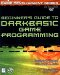Beginners Guide to DarkBASIC Game Programming (Premier Press Game Development)
ISBN: 1592000096
EAN: 2147483647
Year: 2002
Pages: 203

Similar book on Amazon